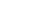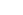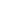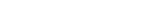How Cheenta works to ensure student success?
Explore the Back-Story

# Group with Quotient : TIFR GS 2018 Part A Problem 16# Understand the problem

Let G be a finite group with a normal subgroup H such that G/H has
order 7. ThenH × G/H.

Hint 1
This is also an interesting question. First of all we need to understand something in general.
If G is a finite group and H Δ G. So Consider the quotient group G/H.
Observe the following!
• Lemma: If G ≈ H x G/H , then G/H is isomorphic to a normal subgroup of G. [Consider the projection homomorphism of G to (H,1) which contains G/H as the kernel.]
• But in general G/H is not even a subgroup of G.
We will illustrate this by giving a simple example.
Hint 2
• Naturally we took the group (Z,+) and we know all the subgroups of Z are nZ ,which are normal subgroups as Z is an abelian group.
• Consider the quotient group Z/nZ.We know that this is not even isomorphic to a subgroup of Z.
• Hence comes our counter-example.
• G=Z, H=7Z. G/H =Z/7Z. But G is not isomorphic to 7Z x Z/7Z as Z/7Z is not isomorphic to a subgroup of Z.
• Hence the answer is False.
Hint 3
1. But we will give an example where the given statement is also False.
• Consider the Dihedral group on n elementsas a subgroup of O(2) [The orthogonal group in.] There is a homomorphism (Determinant) from O(2) → {-1,1},whose kernel is SO(2).
• Hence consider the homomorphism from→ {-1,1} formed by the composition of inclusion homomorphism and the determinant homomorphism.
• Observe that the Kernel of the above defined homomorphism is the Rotation Group of angle 2 π /n and the Quotient Group is the Reflection Group around a specific line(?)[Which is essentially Z/2Z.]
• But observe that Dn is not isomorphic to Rotation Group of angle 2 π /n x Z/2Z .[As there is an interaction between rotation and reflection..]
Hint 4
1. Prove that the finite subgroups of the group of rigid body motion are only
• Rotation Group of Angle 2 π /n for all n in N.
• Dihedral Group# Connected Program at Cheenta

The higher mathematics program caters to advanced college and university students. It is useful for I.S.I. M.Math Entrance, GRE Math Subject Test, TIFR Ph.D. Entrance, I.I.T. JAM. The program is problem driven. We work with candidates who have a deep love for mathematics. This program is also useful for adults continuing who wish to rediscover the world of mathematics.

# Understand the problem

Let G be a finite group with a normal subgroup H such that G/H has
order 7. ThenH × G/H.

Hint 1
This is also an interesting question. First of all we need to understand something in general.
If G is a finite group and H Δ G. So Consider the quotient group G/H.
Observe the following!
• Lemma: If G ≈ H x G/H , then G/H is isomorphic to a normal subgroup of G. [Consider the projection homomorphism of G to (H,1) which contains G/H as the kernel.]
• But in general G/H is not even a subgroup of G.
We will illustrate this by giving a simple example.
Hint 2
• Naturally we took the group (Z,+) and we know all the subgroups of Z are nZ ,which are normal subgroups as Z is an abelian group.
• Consider the quotient group Z/nZ.We know that this is not even isomorphic to a subgroup of Z.
• Hence comes our counter-example.
• G=Z, H=7Z. G/H =Z/7Z. But G is not isomorphic to 7Z x Z/7Z as Z/7Z is not isomorphic to a subgroup of Z.
• Hence the answer is False.
Hint 3
1. But we will give an example where the given statement is also False.
• Consider the Dihedral group on n elementsas a subgroup of O(2) [The orthogonal group in.] There is a homomorphism (Determinant) from O(2) → {-1,1},whose kernel is SO(2).
• Hence consider the homomorphism from→ {-1,1} formed by the composition of inclusion homomorphism and the determinant homomorphism.
• Observe that the Kernel of the above defined homomorphism is the Rotation Group of angle 2 π /n and the Quotient Group is the Reflection Group around a specific line(?)[Which is essentially Z/2Z.]
• But observe that Dn is not isomorphic to Rotation Group of angle 2 π /n x Z/2Z .[As there is an interaction between rotation and reflection..]
Hint 4
1. Prove that the finite subgroups of the group of rigid body motion are only
• Rotation Group of Angle 2 π /n for all n in N.
• Dihedral Group# Connected Program at Cheenta

The higher mathematics program caters to advanced college and university students. It is useful for I.S.I. M.Math Entrance, GRE Math Subject Test, TIFR Ph.D. Entrance, I.I.T. JAM. The program is problem driven. We work with candidates who have a deep love for mathematics. This program is also useful for adults continuing who wish to rediscover the world of mathematics.

# Similar Problems

This site uses Akismet to reduce spam. Learn how your comment data is processed.

### Knowledge Partner